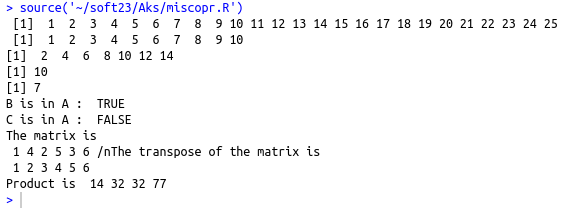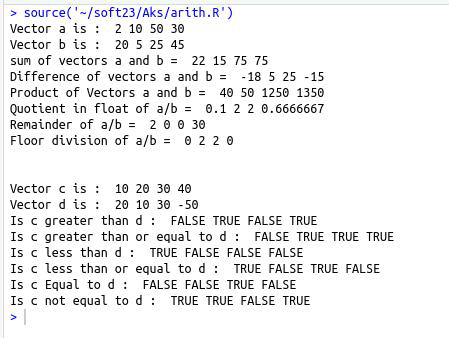• #5, First Floor, 4th Street Dr. Subbarayan Nagar Kodambakkam, Chennai-600 024 Landmark : Samiyar Madam
• pro@slogix.in
• +91- 81240 01111

#### Social List

##### What are all the Operators available in R?
###### Description

To understand different operator and its function available in R.

###### Process
• Types of arithmetic operators
• Arithmetic operators
• Relational operators
• Logical operators
• Assignment operators
• Miscellaneous operators

Arithmetic operators :

• + – Adds the element of a vector to the corresponding element of the another vector
• – – Subtracts the elements of second vector from the corresponding element of first vector
• * – Element by element multiplication
• / – Element by element Float division result – quotient
• %% – Element by element division with remainder as result
• %/% – Element by element floor division result-quotient
• ^ – First vector raised to the exponent of second vector

Relational Operators :
> , >= , < , <= , == , !=
Logical operators :

• & – Element wise logical AND
• | – Element wise Logical OR
• ! – Not of an element
• && – Logical AND of first element of vectors
• || – Logical OR of first element of vectors

Assignment Operators :
, ->> , =
Miscellaneous Operators :
: – Slicing operator

• %in% – Membership operator
• %*% – Product of matrix and its transpose
###### Sapmle Code

Source code :(Arithmetic and relational operators)

#Arithmetic operators

a=c(2,10,50,30)

b=c(20,5,25,45)

cat(“Vector a is : “,a,”\n”)

cat(“Vector b is : “,b,”\n”)

cat(“sum of vectors a and b = “,a+b,”\n”)

cat(“Difference of vectors a and b = “,a-b,”\n”)

cat(“Product of Vectors a and b = “,a*b,”\n”)

cat(“Quotient in float of a/b = “,a/b,”\n”)

cat(“Remainder of a/b = “,a%%b,”\n”)

cat(“Floor division of a/b = “,a%/%b,”\n”)

#Relational Operators

c=c(10,20,30,40)

d=c(20,10,30,-50)

cat(“\n\nVector c is : “,c,”\n”)

cat(“Vector d is : “,d,”\n”)

cat(“Is c greater than d : “,c>d,”\n”)

cat(“Is c greater than or equal to d : “,c>=d,”\n”)

cat(“Is c less than d : “,c<d,”\n”)

cat(“Is c less than or equal to d : “,c<=d,”\n”)

cat(“Is c Equal to d : “,c==d,”\n”)

cat(“Is c not equal to d : “,c!=d,”\n”)

Source code :(Logical operators)

#logical operators

a=c(TRUE,77,0,-80)

b=c(0,55,FALSE,90)

cat(“Vector a is : “,a,”\n”)

cat(“vector b is : “,b,”\n”)

cat(“Element wise Logical AND b/w a and b is “,a&b,”\n”)

cat(“Element wise Logical OR b/w a and b is “,a|b,”\n”)

cat(“Logical NOT of b is “,!b,”\n”)

cat(“Logical AND b/w a and b is “,a&&b,”\n”)

cat(“Logical OR b/w a and b is “,a||b,”\n”)

Source code :(Assignment operators)

#ASSIGNMENT OPERATORS

a=5

b<-10

x<d

25->>e

#c<<-40

cat(“Value of A is “,a,”\n”)

cat(“Value of B is “,b,”\n”)

cat(“Value of X is “,x,”\n”)

cat(“Value of D is “,d,”\n”)

cat(“Value of E is “,e,”\n”)

#cat(“Value of c is :”,c,”\n”)

Source code :(Miscellaneous operators)

#The colon operator

v
print(v)

print(v[1:10])

#membership operator

a=c(2,4,6,8,10,12,14)

b=10

c=7

print(a)

print(b)

print(c)

cat(“B is in A : “,b%in%a,”\n”)

cat(“C is in A : “,c%in%a,”\n”)

#produt of matrix and transpose of matrix

M=matrix(c(1:6),nrow=2,ncol=3,byrow = TRUE)

N=M%*%t(M)

cat(“The matrix is\n”,M,”/n”)

cat(“The transpose of the matrix is\n”,t(M),”\n”)

cat(“Product is “,N)

###### Screenshots